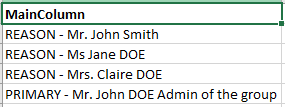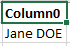# DT.Select(sampleText LIKE '%ColumnName%')

Hello,
I have 2 datatables [dt1] & [dt2]. Both datatables have a single column. The dt2 column name is “Column0”
I’m looping with For Each Row on [dt1]
Within the loop I have to check the next condition :
dt2.Select(“'” + CurrentRow(0).ToString + “’ LIKE ‘%Column0%’”).Count > 0
CurrentRow(0).ToString might be “A B C D”
Column0 might be “C D”
This condition is not returning true, but if I try with a value from the Column0 of dt2 it works while it matches with the value in dt1
Is the condition well declared?

we would recommend to share with us a few sample data of dt1, dt2

The case has the potential to get solved with different approaches

@SONYC If I understand correctly, you want to determine if the field from the current row in dt1 (Let’s say its “A”) is contained in any of the data in dt2’s Column0, right?

So if dt1 CurRow = “A” then False, but f its “D” then True. Is this a fair assumption?

If so, then you can use the “Output Data Table” Activity to put data to a String variable, then see if the string contains the dt1 value. I.E
Before the loop:
Output Data Table to string strDatTab

Within the loop:
bolExists = strDatTab.Contains(CurrentRow(0).ToString)

Actually, I have a main datatable which I called [dt1].
This time I will call it [dtMain]. In this DT we might have several hundred rows.
The information contained in the ‘MainColumn’ of this [dtMain] in the next format:I have 5 other datatables containing information in the next format:I need to check the presence of all the names from the 5 small DTs in the [dtMain] and at the end populate another DT with the names from [dtMain] that were not found in any of the 5 small DTs.

For this I loop on [dtMain] and for each row, I check to see if any value from [dt1] is contained in the CurrentRow and if the value is found I set up a true boolean. I do this check for all the 5 DTs inside the ‘For Each Row’ loop and at the end if the ‘Row’ didn’t contain any of the values form any small DT, I populate a final DT with the the specific ‘Row’.
That is my approach considering the need.

In my conditions

dt2.Select(“‘” + CurrentRow(0).ToString + “’ LIKE ‘%Column0%’”).Count > 0
dt3.Select(“’” + CurrentRow(0).ToString + “’ LIKE ‘%Column0%’”).Count > 0

I want to check if the name “Jane DOE” is contained in the value of the CurrentRow of the [dtMain] and populat the final DT only with the rows not containing the info from any DT.

I don’t believe what you are trying to do is possible with the Select() function. An alternative solution is to use LINQ instead:

``````dt2.AsEnumerable.Any(Function(x) CurrentRow(0).ToString.Contains(x("Column0").ToString))
``````

Thank you very much! It fits perfectly.

What would be the expression if I want to retrieve the matching string from [dt2]?

``````matchingRows = dt2.AsEnumerable().Where(Function(row) CurrentRow(0).ToString().Contains(row("Column0").ToString)).ToArray
``````

Where matchingRows is an array of DataRow.

Thank you.
Knowing that the datarow contains just one item, I would like to log the value of the matching item.

This topic was automatically closed 3 days after the last reply. New replies are no longer allowed.